Home | | Physics 12th Std | Torque Acting on a Bar Magnet in Uniform Magnetic Field

# Torque Acting on a Bar Magnet in Uniform Magnetic Field

Physics : Magnetism and Magnetic Effects of Electric Current: Torque Acting on a Bar Magnet in Uniform Magnetic Field and Solved Example Problems

TORQUE ACTING ON A BAR MAGNET IN UNIFORM MAGNETIC FIELD

Consider a magnet of length 2l of pole strength qm kept in a uniform magnetic field B as shown in Figure 3.19. Each pole experiences a force of magnitude qmB but acts in opposite direction. Therefore, the net force exerted on the magnet is zero, so that there is no translatory motion. These two forces constitute a couple (about midpoint of bar magnet) which will rotate and try to align in the direction of the magnetic field.Adding equations (3.23) and (3.24), we get the net force acting on the dipole asThis implies, that the net force acting on the dipole is zero, but forms a couple which tends to rotate the bar magnet clockwise (here) in order to align it along.

The moment of force or torque experienced by north and south pole about point O isBy using right hand cork screw rule, we conclude that the total torque is pointing into the paper. Since the magnitudesthe magnitude of total torque about point OEXAMPLE 3.7

Show the time period of oscillation when a bar magnet is kept in a uniform magnetic field isin second, where I represents moment Pm of inertia of the bar magnet, pm is the magnetic moment and is the magnetic field.

Solution

The magnitude of deflecting torque (the torque which makes the object rotate) acting on the bar magnet which will tend to align the bar magnet parallel to the direction of the uniform magnetic fieldisThe magnitude of restoring torque acting on the bar magnet can be written asUnder equilibrium conditions, both magnitude of deflecting torque and restoring torque will be equal but act in the opposite directions, which meansThe negative sign implies that both are in opposite directions. The above equation can be written asThis is non-linear second order homogeneous differential equation. In order to make it linear, we use small angle approximation as we did in XI volume II (Unit 10 – oscillations, Refer section 10.4.4) i.e., sin, we getThis linear second order homogeneous differential equation is a Simple Harmonic differential equation. Therefore,

Comparing with Simple Harmonic Motion (SHM) differential equationwhere ω is the angular frequency of the oscillation.where, BH is the horizontal component of Earth’s magnetic field.

## 1. Potential energy ofa bar magnet in a uniform magnetic field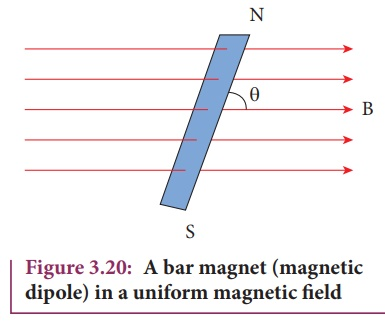When a bar magnet (magnetic dipole) of dipole momentm is held at an angle θ with the direction of a uniform magnetic field, as shown in Figure 3.20 the magnitude of the torque acting on the dipole isIf the dipole is rotated through a very small angular displacement dθ against the torque τB at constant angular velocity, then the work done by external torque () for this small angular displacement is given by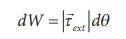The bar magnet has to be moved at constant angular velocity, which implies that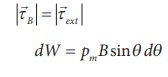Total work done in rotating the dipole from θʹ to θ isThis work done is stored as potential energy in bar magnet at an angle θ when it is rotated from θʹ to θ and it can be written asIn fact, the equation (3.26) gives the difference in potential energy between the angular positions θʹ and θ. We can choose the reference point θʹ = 90o, so that second term in the equation becomes zero and the equation (3.26) can be written as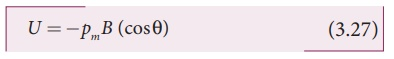The potential energy stored in a bar magnet in a uniform magnetic field is given byCase 1

(i) If θ = 0º, then

U = − pm B (cos0 º ) = − pm B

(ii) If θ = 180 º, then

U= − pm B (cos180 º) = pm B

We can infer from the above two results, the potential energy of the bar magnet is minimum when it is aligned along the external magnetic field and maximum when the bar magnet is aligned anti-parallel to external magnetic field.

EXAMPLE 3.8

Consider a magnetic dipole which on switching ON external magnetic field orient only in two possible ways i.e., one along the direction of the magnetic field (parallel to the field) and another anti-parallel to magnetic field. Compute the energy for the possible orientation. Sketch the graph.

Solution

Letm  be the dipole and before switching ON the external magnetic field, there is no orientation. Therefore, the energy U = 0.

As soon as external magnetic field is switched ON, the magnetic dipole orient parallel (θ = 0º) to the magnetic field with energy,

Uparallel = Uminimum = − pm B cos0

Uparallel = − pm B

since cos 0º = 1

Otherwise, the magnetic dipole orients anti-parallel (θ = 180º) to the magnetic field with energy,

Uanti− parallel = U maximum =− pmB cos180

U anti−parallel = pmB

since cos 180º = -1## 2. Tangent law andTangent Galvanometer

Tangent Galvanometer (Figure 3.21) is a device used to measure very small currents. It is a moving magnet type galvanometer. Its working is based on tangent law.### Tangent law

When a magnetic needle or magnet is freely suspended in two mutually perpendicular uniform magnetic fields, it will come to rest in the direction of the resultant of the two fields.

Let B be the magnetic field produced by passing current through the coil of the tangent Galvanometer and BH be the horizontal component of earth’s magnetic field. Under the action of two magnetic fields, the needle comes to rest making angle θ with BH, such that### Construction

Tangent Galvanometer (TG) consists of copper coil wounded on a non-magnetic circular frame. The frame is made up of brass or wood which is mounted vertically on a horizontal base table (turn table) with three levelling screws as shown in Figure 3.22. The TG is provided with two or more coils of different number of turns. Most of the equipment we use in laboratory consists of 2 turns, 5 turns and 50 turns which are of different thickness and are used for measuring currents of different strengths.

At the center of turn table, a small upright projection is seen on which compass box (also known as magnetometre box) is placed. Compass box consists of a small magnetic needle which is pivoted at the center, such that arrangement shows the center of both magnetic needle and circular coil exactly coincide. A thin aluminium pointer is attached to the magnetic needle normally and moves over circular scale. The circular scale is divided into four quadrants and graduated in degrees which are used to measure the deflection of aluminium pointer on a circular degree scale. In order to avoid parallax error in measurement, a mirror is placed below the aluminium pointer.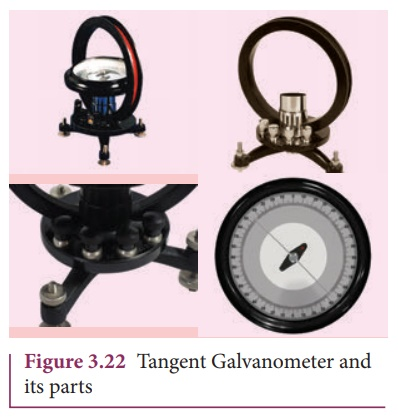Precautions

1.     All the nearby magnets and magnetic materials are kept away from the instrument.

2. Using spirit level, the levelling screws at the base are adjustedso that the small magnetic needle is exactly horizontal and also coil (mounted on the frame) is exactly vertical.

3. The plane of the coil is kept parallel to the small magnetic needle by rotating the coil about its vertical axis. So, the coil remains in magnetic meridian.4. The compass box (as shown in Figure 3.23) is rotated such that the pointer reads 0º – 0 º

### Theory

The circuit connection for Tangent Galvanometer (TG) experiment is shown in Figure 3.24. When no current is passed through the coil, the small magnetic needle lies along horizontal component of Earth’s magnetic field. When the circuit is switched ON, the electric current will pass through the circular coil and produce magnetic field. The magnetic field produced due to the circulatory electric current is discussed (in section 3.8.3). Now there are two fields which are acting mutually perpendicular to each other. They are:

(1) the magnetic field (B) due to the electric current in the coil acting normal to the plane of the coil.

(2) the horizontal component of Earth’s magnetic field (BH)Because of these crossed fields, the pivoted magnetic needle deflects through an angle θ. From tangent law (equation 3.29),

B = BH tan θ

When an electric current is passed through a circular coil of radius R having N turns, the magnitude of magnetic field at the center isFrom equation (3.29)  and equation (3.30), we getThe horizontal component of Earth’s magnetic field can be determined asEXAMPLE 3.9

A coil of a tangent galvanometer of diametre 0.24 m has 100 turns. If the horizontal component of Earth’s magnetic field is 25 × 10-6 T then, calculate the current which gives a deflection of 60º.

Solution

The diameter of the coil is 0.24 m.

Therefore, radius of the coil is 0.12 m.

Number of turns is 100 turns. Earth’s magnetic field is 25 x 10-6 T

Deflection is

θ=60 º tan 60 º = √3 = 1.732I = 0.082 A

Study Material, Lecturing Notes, Assignment, Reference, Wiki description explanation, brief detail
12th Physics : Magnetism and Magnetic Effects of Electric Current : Torque Acting on a Bar Magnet in Uniform Magnetic Field |